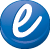(800)444-4287
Mon - Fri, 8:00AM - 4:30PM PTThis is a downloadable product.
•Sample Pages
SKU: 8355-EB

# Power Practice: Fractions and Decimals, Gr. 3-4, eBook

\$4.99
• Product Type: eWorkbook
• UPC:
• ISBN: 978-1-59198-934-9
• DESCRIPTION
• REVIEWS
• specifications
DESCRIPTION

Reproducible Activities for Targeted Practice

• Comparing fractions
• Adding and subtracting mixed numbers
• Multiplying and dividing fractions
• Finding the least common denominator
• Comparing decimals
• Rounding decimals
• Multiplying and dividing decimals
• Converting fractions to decimals

This Power Practice series reinforces the specific math and language arts skills that students must master in order to experience success on standardized tests. Each book explores one or two concepts in depth by presenting them in a logical, sequenced progression of smaller objectives.

Fractions and Decimals 3-4 provides problem-solving activities that allow students to apply what they have learned and practice critical thinking skills. Skills covered include the following:

• comparing fractions and ordering fractions
• changing improper fractions to mixed numbers
• matching fractions and decimals
• comparing and ordering decimals
• rounding decimals